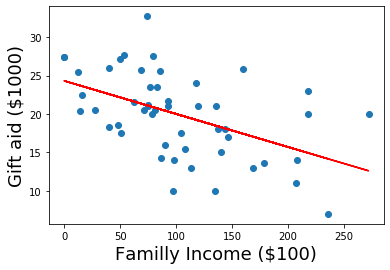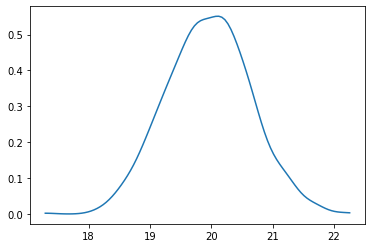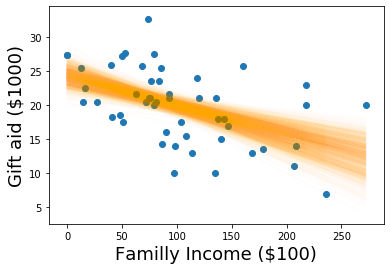In :
import numpy as np
import pandas as pd
from scipy.stats import norm as normal
from scipy.optimize import minimize
import bplot as bp
import patsy


# Simple Linear Regression¶

In :
def ll_normal(beta, yX):
y = yX[:, 0]
X = yX[:, 1:]
N = X.shape
mu = np.full(N, np.nan)
for n in range(N):
mu[n] = np.sum(X[n, :] * beta)
d = y - mu
return np.sum(d * d)

def optim(data, initval = None):
k = data.shape - 1
if not initval:
initval = np.random.normal(size = k)
return minimize(ll_normal, initval, args=(data), method="BFGS")['x']

def bootstrap(data, R, fun):
N, k = data.shape
k -= 1
thetas = np.full((R, k), np.nan)
for r in range(R):
idx = np.random.choice(N, N, replace=True)
thetas[r, :] = fun(data[idx, :])
return thetas

R = 1001

In :
df = pd.read_csv("https://raw.githubusercontent.com/roualdes/data/master/elmhurst.csv")

In :
# Explanitory variable (x-axis) is family income, and (y-axis) is the resulting gift aid.

# We are making predictions on gift aid given family income.

In :
X = patsy.dmatrix("~ family_income", data=df)

In :
betahat = minimize(ll_normal, np.random.normal(size=2), args=yX, method="BFGS")['x']

In :
bp.point(df["family_income"], df["gift_aid"])
bp.labels(x = "Familly Income ($100)", y = "Gift aid ($1000)", size = 18)
# betahat * df['family_income']
bp.line(df['family_income'], betahat + betahat * df['family_income'], color = 'red')

Out:
[<matplotlib.lines.Line2D at 0x11d1adc10>]In :
# For every $1000 increase in family income, we expect gift aid to decrease by ~$40
betahat #24.32 + -0.04(Xn)

Out:
array([24.31933199, -0.04307168])
In :
u = 24.32 + -0.04 * (100) # the units are $1000, so really we are inputing$100,000

# How Edward did it
np.sum(betahat * np.asarray([1, 100]))

Out:
20.012163905185982
In :
betas = bootstrap(yX, R, optim)

/Users/ez/venvs/py3/lib/python3.7/site-packages/scipy/optimize/optimize.py:1048: RuntimeWarning: divide by zero encountered in double_scalars
rhok = 1.0 / (numpy.dot(yk, sk))
/Users/ez/venvs/py3/lib/python3.7/site-packages/scipy/optimize/optimize.py:1048: RuntimeWarning: divide by zero encountered in double_scalars
rhok = 1.0 / (numpy.dot(yk, sk))
/Users/ez/venvs/py3/lib/python3.7/site-packages/scipy/optimize/optimize.py:1048: RuntimeWarning: divide by zero encountered in double_scalars
rhok = 1.0 / (numpy.dot(yk, sk))
/Users/ez/venvs/py3/lib/python3.7/site-packages/scipy/optimize/optimize.py:1048: RuntimeWarning: divide by zero encountered in double_scalars
rhok = 1.0 / (numpy.dot(yk, sk))

In :
"""
If a family aint' got no money, we are 80% confident the gift aid will be between 22.82 and 25.98 thousands
of dollars

For every $1000 dollar increase in income, we are 80% confident that there is decrease by between 0.029 to 0.06 in$1000 dollars of gift aid
"""
np.percentile(betas, [10, 90], axis=0).T

Out:
array([[22.73844549, 25.9926342 ],
[-0.05997412, -0.02882947]])
In :
giftaidhat = np.full(R, np.nan)
for r in range(R):
giftaidhat[r] = np.sum(np.asarray([1,100]) * betas[r, :])

In :
# vectorized
gitfaidhat = np.sum(np.asarray([1, 100] * betas), axis=1)

In :
bp.density(giftaidhat)

Out:
[<matplotlib.lines.Line2D at 0x11d050d90>]In :
"""
we are 80% confident that as the family income reaches $100,000 the gift aid will be between 19.17 and 20.81$1000s of dollars

"""


Out:
array([19.05822484, 20.80087528])
In :
giftaidhat = np.full(R, np.nan)
for r in range(R):
bp.line(df['family_income'], betas[r, 0] + betas[r, 1] * df['family_income'],
color="orange", alpha=0.01)
giftaidhat[r] = np.sum(np.asarray([1,100]) * betas[r, :])

bp.labels(x = "Familly Income ($1000)", y = "Gift aid ($1000)", size = 18)

<matplotlib.axes._subplots.AxesSubplot at 0x11f44de90>